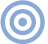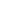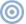# Features

## An Amplifier Listening Test

It is always a matter of great interest when a difficult question, in this case the audibility of differences between amplifiers, is put to an empirical test. When the question is tested by such intelligent, knowledgeable, and unbiased investigators as John Atkinson and Will Hammond (see the July issue of Stereophile, Vol.12 No.7, p.5, the interest is even greater. Unfortunately, when the test turns out to have been flawed by errors in design and in use of statistics, as was the case here, the disappointment is also even greater.

In this article (footnote 1), we first explain the statistical errors and present a reanalysis using the correct statistics. We then report a study that corrects some of the flaws we find in the Stereophile study and shows that the audible differences between the two amplifiers are much greater than the original study implied. Finally, we conclude with some brief reflections on approaches to investigating the listening qualities of amplifiers.

Two statistical problems in the Stereophile listening test completely invalidate the conclusions. The first is JA's inappropriate application of the chi-square test—but before discussing the flaws in the use of the test, we should briefly explain what this test is. In statistics there are two kinds of data analysis: descriptive and inferential. A descriptive statistic is exactly that: a number or set of numbers derived in some way from the data to give a succinct description of an important aspect of the data.

For example, the various kinds of average, such as mean or median, are descriptive statistics that express the central tendency of the data. Descriptive statistics are very important because we usually cannot digest or remember an entire data set and thus need some smaller set of numbers that adequately characterize the important features of the set. That is why we have such things as batting averages for baseball players. A full set of data listing times at bat and hits (in what inning, against what pitcher, etc.) would tell a lot more about the player, but that's too much to remember unless you happen to be an idiot savant or an obsessive fan. The single number, the batting average, is an adequate characterization of the batter's ability for most purposes.

An inferential statistic is fundamentally different from a descriptive statistic. It attempts to go beyond the given data to infer whether a pattern of data is a result of some underlying cause or whether the pattern is merely the result of chance. A deep insight about the nature of truth is embodied in inferential statistics. The underlying logic of inferential statistics recognizes that absolute certainty can never be obtained; the statistics are designed only to estimate the probability that the observed pattern is due to chance. Thus, using inferential statistics we can calculate that the probability of a pattern resulting from chance is less than some amount, say, less than 1 in 20 or 1 in 100. What such a statement means is that, by our best estimate, chance alone would produce the pattern less than once in 20 or once in 100 tries.

The chi-square is one such inferential statistic. We cannot take the space here to describe how it works, but suffice it to say that the test depends on a number of reasonable mathematical assumptions to estimate the probability that a pattern of data is simply a result of chance. If these assumptions are violated, the estimate is highly questionable.

One of these assumptions, and a very important one, is that observations that enter into the calculation of chi-square must be independent. This is the assumption that was violated in the Stereophile test. It is incorrect to treat each response of a subject as a separate, independent observation for chi-square testing of significance. Why? Because each observer's seven responses are influenced by that observer's biases, accuracy, and any other inherent characteristics he or she may have. The chi-square test looks to see if the obtained results deviate from chance expectations. If the entries come in nonindependent "clumps," then the test is likely to show that the results differ from chance only because of the non-independence of the samples, not because of any real differences.

To put this matter in more intuitive terms, counting every observer response as an independent observation is very much like letting people vote more than once in an election. If everyone votes, say, seven times, the winner will still get the same proportion of the votes, but the result will seem more impressive and contrary-to-chance than it really was. The inflation of votes creates a serious statistical problem because the inferential techniques take advantage of the fact that, as observations increase, random events tend to average out.

As numbers increase, for example, flips of fair coins tend to approach a 50:50 ratio of heads and tails. In ten flips, a finding of 60% heads would not be very surprising, but if the 60% held up over 1000 flips, the probability that the coin was fair would be extremely low. Likewise, a small percentage difference from chance is much less remarkable with 505 observations (the actual number of listeners in the Stereophile study) than with 3530 (the total number of responses in the study: 505 listeners times 7 judgments minus five missing responses). It is 505, not 3530, that is the correct number for estimation of randomness in this case. Consequently, the observed findings are less reliable than was thought. How much less, we will consider later.ARTICLE CONTENTSX